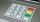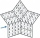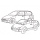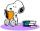# Natural numbers + Pythagoras contest - math problems

#### Number of problems found: 8

• Phone numberIvan's phone number ends with a four-digit number: When we subtract the first from the fourth digit of this four-digit number, we get the same number as when we subtract the second from the third digit. If we write the four-digit number from the back and
• Five-digit numberAnna thinks of a five-digit number that is not divisible by three or four. If he increments each digit by one, it gets a five-digit number that is divisible by three. If he reduces each digit by one, he gets a five-digit number divisible by four. If it sw
• All pairsDetermine all pairs (m, n) of natural numbers for which is true: m s (n) = n s (m) = 70, where s (a) denotes the digit sum of the natural number a.
• Apples and pearsApples cost 50 cents piece, pears 60 cents piece, bananas cheaper than pears. Grandma bought 5 pieces of fruit. There was only one banana, and I paid 2 euros 75 cents. How many apples and how many pears?
• Two cars on ringThere were two cars on the round track (ring) in the adjacent tracks, the first car in the inner track, the second in the outer track. Both cars started at the same time from one starting track. The first toy car drove every four laps simultaneously as th
• Self-counting machineThe self-counting machine works exactly like a calculator. The innkeeper wanted to add several three-digit natural numbers on his own. On the first attempt, he got the result in 2224. To check, he added these numbers again and he got 2198. Therefore, he a
• Equation algebraogramSolve the equation: oco + ivo = cita How much has the task of solutions?
• Alarm clockThe old watchmaker has a unique digital alarm in its collection that rings whenever the sum of digits of the alarm is equal to 21. Find out when the alarm clock will ring. What is their number? List all options . ..

We apologize, but in this category are not a lot of examples.
Do you have an exciting math question or word problem that you can't solve? Ask a question or post a math problem, and we can try to solve it.

We will send a solution to your e-mail address. Solved examples are also published here. Please enter the e-mail correctly and check whether you don't have a full mailbox.

Natural numbers - math problems. Pythagoras contest - math problems.